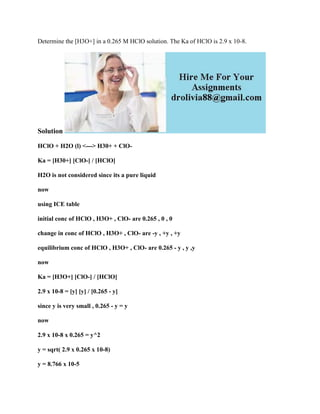Ce diaporama a bien été signalé.
Le téléchargement de votre SlideShare est en cours. ×

# Determine the -H3O+- in a 0-265 M HClO solution- The Ka of HClO is 2-9.docx

Publicité
Publicité
Publicité
Publicité
Publicité
Publicité
Publicité
Publicité
Publicité
Publicité
PublicitéChargement dans…3
×

## Consultez-les par la suite

1 sur 2 Publicité

# Determine the -H3O+- in a 0-265 M HClO solution- The Ka of HClO is 2-9.docx

Determine the [H3O+] in a 0.265 M HClO solution. The Ka of HClO is 2.9 x 10-8.
Solution
HClO + H2O (l) <---> H30+ + ClO-
Ka = [H30+] [ClO-] / [HClO]
H2O is not considered since its a pure liquid
now
using ICE table
initial conc of HClO , H3O+ , ClO- are 0.265 , 0 , 0
change in conc of HClO , H3O+ , ClO- are -y , +y , +y
equilibrium conc of HClO , H3O+ , ClO- are 0.265 - y , y ,y
now
Ka = [H3O+] [ClO-] / [HClO]
2.9 x 10-8 = [y] [y] / [0.265 - y]
since y is very small , 0.265 - y = y
now
2.9 x 10-8 x 0.265 = y^2
y = sqrt( 2.9 x 0.265 x 10-8)
y = 8.766 x 10-5
now
[H30+] = y = 8.766 x 10 -5 M
.

Determine the [H3O+] in a 0.265 M HClO solution. The Ka of HClO is 2.9 x 10-8.
Solution
HClO + H2O (l) <---> H30+ + ClO-
Ka = [H30+] [ClO-] / [HClO]
H2O is not considered since its a pure liquid
now
using ICE table
initial conc of HClO , H3O+ , ClO- are 0.265 , 0 , 0
change in conc of HClO , H3O+ , ClO- are -y , +y , +y
equilibrium conc of HClO , H3O+ , ClO- are 0.265 - y , y ,y
now
Ka = [H3O+] [ClO-] / [HClO]
2.9 x 10-8 = [y] [y] / [0.265 - y]
since y is very small , 0.265 - y = y
now
2.9 x 10-8 x 0.265 = y^2
y = sqrt( 2.9 x 0.265 x 10-8)
y = 8.766 x 10-5
now
[H30+] = y = 8.766 x 10 -5 M
.

Publicité
Publicité

### Determine the -H3O+- in a 0-265 M HClO solution- The Ka of HClO is 2-9.docx

1. 1. Determine the [H3O+] in a 0.265 M HClO solution. The Ka of HClO is 2.9 x 10-8. Solution HClO + H2O (l) <---> H30+ + ClO- Ka = [H30+] [ClO-] / [HClO] H2O is not considered since its a pure liquid now using ICE table initial conc of HClO , H3O+ , ClO- are 0.265 , 0 , 0 change in conc of HClO , H3O+ , ClO- are -y , +y , +y equilibrium conc of HClO , H3O+ , ClO- are 0.265 - y , y ,y now Ka = [H3O+] [ClO-] / [HClO] 2.9 x 10-8 = [y] [y] / [0.265 - y] since y is very small , 0.265 - y = y now 2.9 x 10-8 x 0.265 = y^2 y = sqrt( 2.9 x 0.265 x 10-8) y = 8.766 x 10-5
2. 2. now [H30+] = y = 8.766 x 10 -5 M